19.6 Capacitors in series and parallel  (Page 2/2)

 Page 2 / 2

Capacitors in parallel

[link] (a) shows a parallel connection of three capacitors with a voltage applied. Here the total capacitance is easier to find than in the series case. To find the equivalent total capacitance ${C}_{\text{p}}$ , we first note that the voltage across each capacitor is $V$ , the same as that of the source, since they are connected directly to it through a conductor. (Conductors are equipotentials, and so the voltage across the capacitors is the same as that across the voltage source.) Thus the capacitors have the same charges on them as they would have if connected individually to the voltage source. The total charge $Q$ is the sum of the individual charges:

$Q={Q}_{1}+{Q}_{2}+{Q}_{3}.$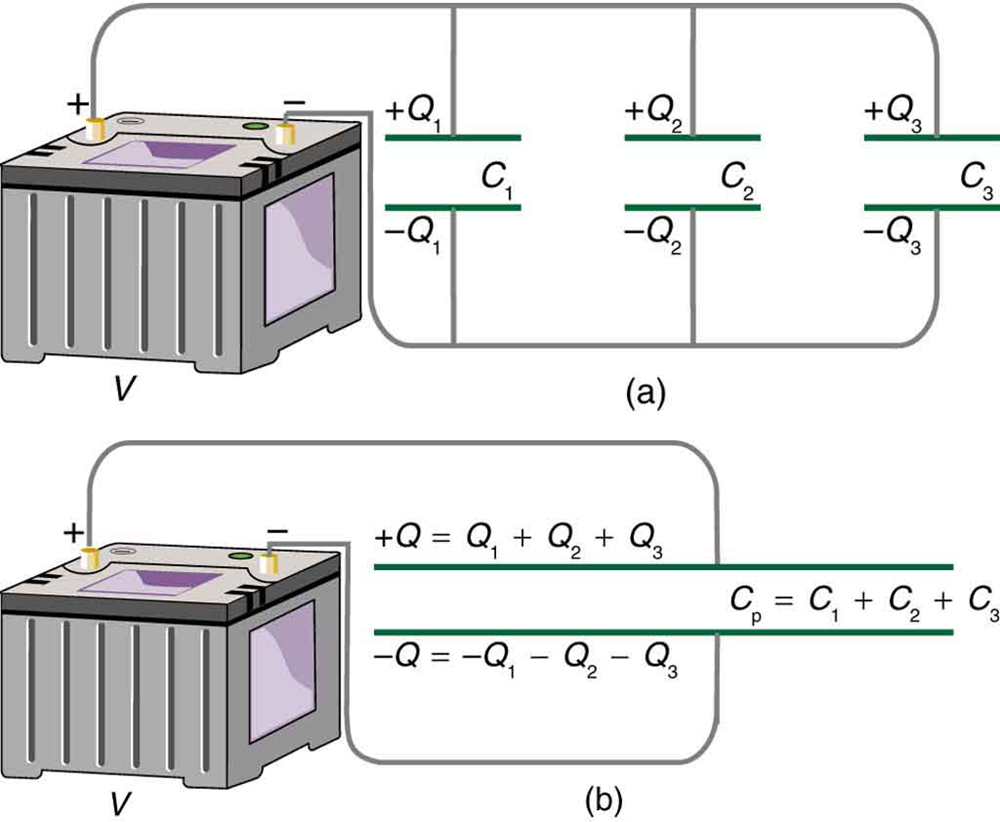(a) Capacitors in parallel. Each is connected directly to the voltage source just as if it were all alone, and so the total capacitance in parallel is just the sum of the individual capacitances. (b) The equivalent capacitor has a larger plate area and can therefore hold more charge than the individual capacitors.

Using the relationship $Q=\text{CV}$ , we see that the total charge is $Q={C}_{\text{p}}V$ , and the individual charges are ${Q}_{1}={C}_{1}V$ , ${Q}_{2}={C}_{2}V$ , and ${Q}_{3}={C}_{3}V$ . Entering these into the previous equation gives

${C}_{\text{p}}V={C}_{1}V+{C}_{2}V+{C}_{3}V.$

Canceling $V$ from the equation, we obtain the equation for the total capacitance in parallel ${C}_{\text{p}}$ :

${C}_{p}={C}_{1}+{C}_{2}+{C}_{3}+\text{.}\text{.}\text{.}.$

Total capacitance in parallel is simply the sum of the individual capacitances. (Again the “ ... ” indicates the expression is valid for any number of capacitors connected in parallel.) So, for example, if the capacitors in the example above were connected in parallel, their capacitance would be

${C}_{\text{p}}=1\text{.}\text{000 µF}+5\text{.}\text{000 µF}+8\text{.}\text{000 µF}=\text{14}\text{.}000 µF.$

The equivalent capacitor for a parallel connection has an effectively larger plate area and, thus, a larger capacitance, as illustrated in [link] (b).

Total capacitance in parallel, ${C}_{\text{p}}$

Total capacitance in parallel ${C}_{\text{p}}={C}_{1}+{C}_{2}+{C}_{3}+\text{.}\text{.}\text{.}$

More complicated connections of capacitors can sometimes be combinations of series and parallel. (See [link] .) To find the total capacitance of such combinations, we identify series and parallel parts, compute their capacitances, and then find the total.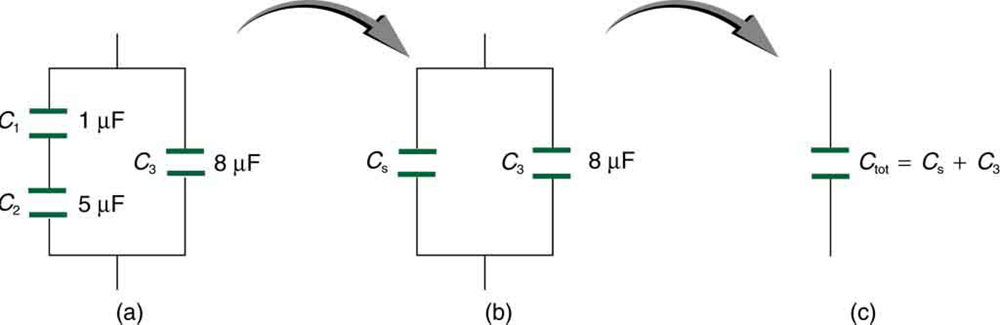(a) This circuit contains both series and parallel connections of capacitors. See [link] for the calculation of the overall capacitance of the circuit. (b) C 1 size 12{ {C} rSub { size 8{1} } } {} and C 2 size 12{ {C} rSub { size 8{2} } } {} are in series; their equivalent capacitance C S size 12{ {C} rSub { size 8{S} } } {} is less than either of them. (c) Note that C S size 12{ {C} rSub { size 8{S} } } {} is in parallel with C 3 size 12{ {C} rSub { size 8{3} } } {} . The total capacitance is, thus, the sum of C S size 12{ {C} rSub { size 8{S} } } {} and C 3 size 12{ {C} rSub { size 8{3} } } {} .

A mixture of series and parallel capacitance

Find the total capacitance of the combination of capacitors shown in [link] . Assume the capacitances in [link] are known to three decimal places ( ${C}_{1}=1.000 µF$ , ${C}_{2}=5.000 µF$ , and ${C}_{3}=8.000 µF$ ), and round your answer to three decimal places.

Strategy

To find the total capacitance, we first identify which capacitors are in series and which are in parallel. Capacitors ${C}_{1}$ and ${C}_{2}$ are in series. Their combination, labeled ${C}_{\text{S}}$ in the figure, is in parallel with ${C}_{3}$ .

Solution

Since ${C}_{1}$ and ${C}_{2}$ are in series, their total capacitance is given by $\frac{1}{{C}_{\text{S}}}=\frac{1}{{C}_{1}}+\frac{1}{{C}_{2}}+\frac{1}{{C}_{3}}$ . Entering their values into the equation gives

$\frac{1}{{C}_{\text{S}}}=\frac{1}{{C}_{1}}+\frac{1}{{C}_{2}}=\frac{1}{1\text{.}\text{000}\phantom{\rule{0.25em}{0ex}}\text{μF}}+\frac{1}{5\text{.}\text{000}\phantom{\rule{0.25em}{0ex}}\text{μF}}=\frac{1\text{.}\text{200}}{\text{μF}}.$

Inverting gives

${C}_{\text{S}}=0\text{.}\text{833 µF}.$

This equivalent series capacitance is in parallel with the third capacitor; thus, the total is the sum

$\begin{array}{lll}{C}_{\text{tot}}& =& {C}_{\text{S}}+{C}_{\text{S}}\\ & =& 0\text{.}\text{833}\phantom{\rule{0.25em}{0ex}}\text{μF}+8\text{.}\text{000}\phantom{\rule{0.25em}{0ex}}\text{μF}\\ & =& 8\text{.}\text{833}\phantom{\rule{0.25em}{0ex}}\text{μF}.\end{array}$

Discussion

This technique of analyzing the combinations of capacitors piece by piece until a total is obtained can be applied to larger combinations of capacitors.

Section summary

• Total capacitance in series $\frac{1}{{C}_{\text{S}}}=\frac{1}{{C}_{1}}+\frac{1}{{C}_{2}}+\frac{1}{{C}_{3}}+\text{.}\text{.}\text{.}$
• Total capacitance in parallel ${C}_{\text{p}}={C}_{1}+{C}_{2}+{C}_{3}+\text{.}\text{.}\text{.}$
• If a circuit contains a combination of capacitors in series and parallel, identify series and parallel parts, compute their capacitances, and then find the total.

Conceptual questions

If you wish to store a large amount of energy in a capacitor bank, would you connect capacitors in series or parallel? Explain.

Problems&Exercises

Find the total capacitance of the combination of capacitors in [link] .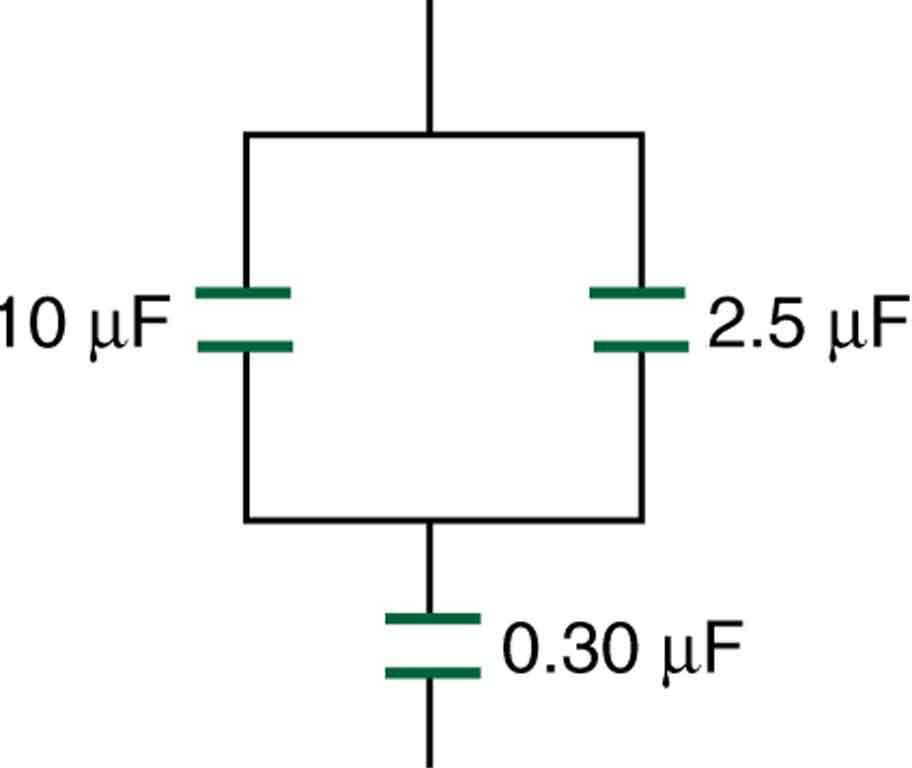A combination of series and parallel connections of capacitors.

$\text{0.293 μF}$

Suppose you want a capacitor bank with a total capacitance of 0.750 F and you possess numerous 1.50 mF capacitors. What is the smallest number you could hook together to achieve your goal, and how would you connect them?

What total capacitances can you make by connecting a $5\text{.}\text{00 µF}$ and an $8\text{.}\text{00 µF}$ capacitor together?

$3\text{.}\text{08 µF}$ in series combination, $\text{13}\text{.}\text{0 µF}$ in parallel combination

Find the total capacitance of the combination of capacitors shown in [link] .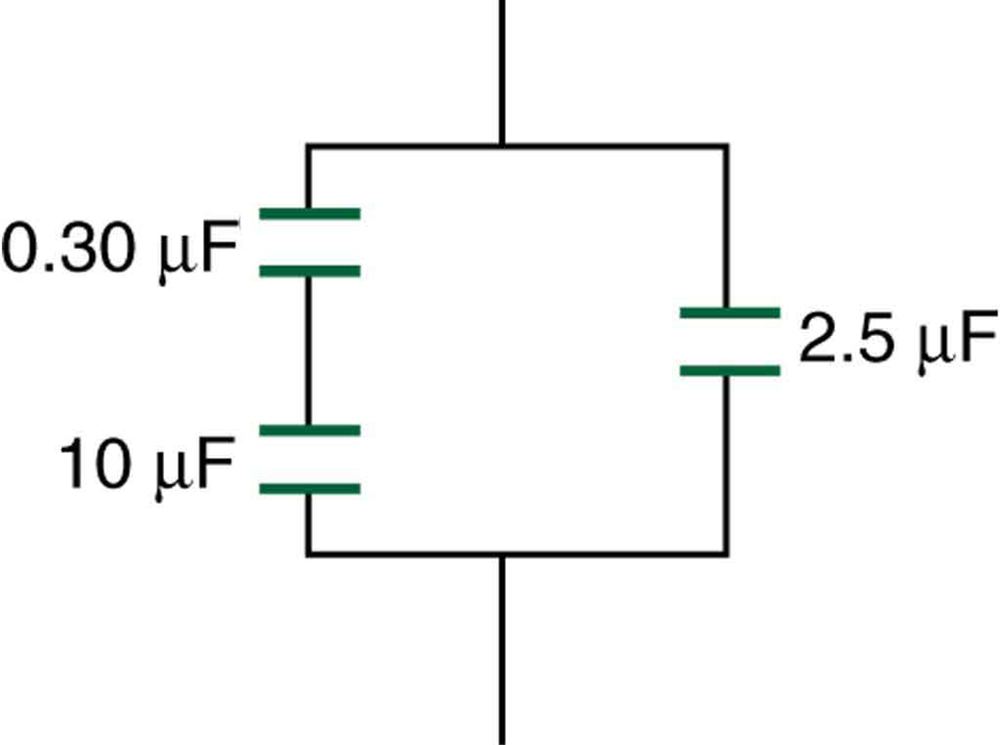A combination of series and parallel connections of capacitors.

$2\text{.}\text{79 µF}$

Find the total capacitance of the combination of capacitors shown in [link] .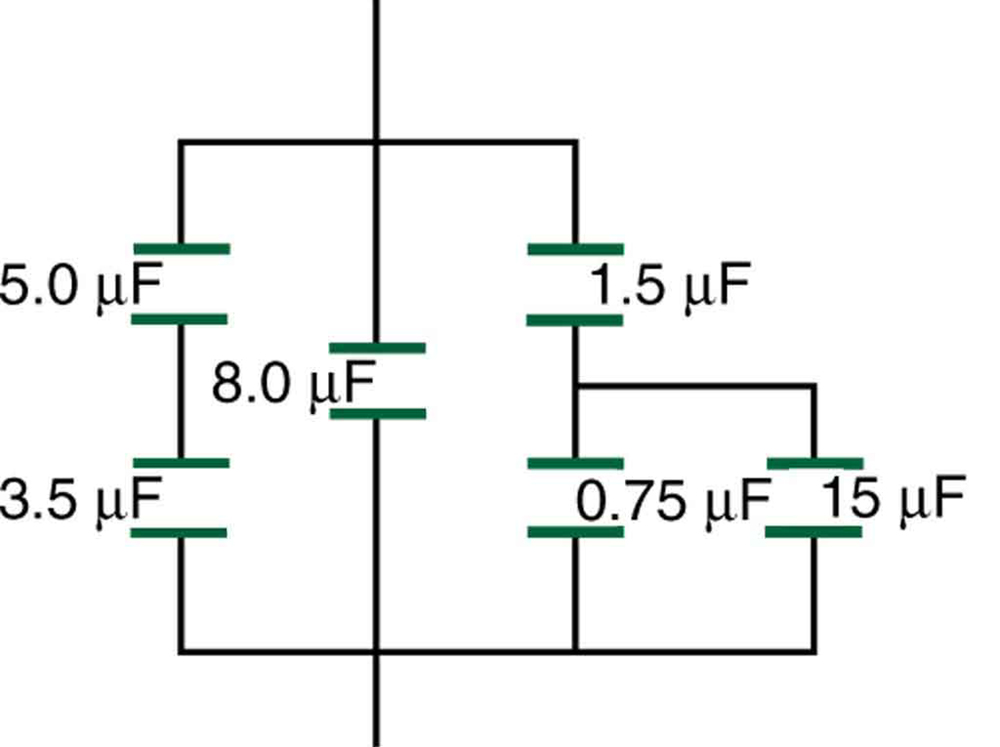A combination of series and parallel connections of capacitors.

Unreasonable Results

(a) An $8\text{.}\text{00 µF}$ capacitor is connected in parallel to another capacitor, producing a total capacitance of $5\text{.}\text{00 µF}$ . What is the capacitance of the second capacitor? (b) What is unreasonable about this result? (c) Which assumptions are unreasonable or inconsistent?

(a) $–3\text{.}\text{00 µF}$

(b) You cannot have a negative value of capacitance.

(c) The assumption that the capacitors were hooked up in parallel, rather than in series, was incorrect. A parallel connection always produces a greater capacitance, while here a smaller capacitance was assumed. This could happen only if the capacitors are connected in series.

what is physics
is the study of matter in relation to energy
Kintu
a submersible pump is dropped a borehole and hits the level of water at the bottom of the borehole 5 seconds later.determine the level of water in the borehole
what is power?
power P = Work done per second W/ t. It means the more power, the stronger machine
Sphere
e.g. heart Uses 2 W per beat.
Rohit
A spherica, concave shaving mirror has a radius of curvature of 32 cm .what is the magnification of a persons face. when it is 12cm to the left of the vertex of the mirror
did you solve?
Shii
1.75cm
Ridwan
my name is Abu m.konnek I am a student of a electrical engineer and I want you to help me
Abu
the magnification k = f/(f-d) with focus f = R/2 =16 cm; d =12 cm k = 16/4 =4
Sphere
A weather vane is some sort of directional arrow parallel to the ground that may rotate freely in a horizontal plane. A typical weather vane has a large cross-sectional area perpendicular to the direction the arrow is pointing, like a “One Way” street sign. The purpose of the weather vane is to indicate the direction of the wind. As wind blows pa
hi
Godfred
what about the wind vane
Godfred
If a prism is fully imersed in water then the ray of light will normally dispersed or their is any difference?
the same behavior thru the prism out or in water bud abbot
Ju
If this will experimented with a hollow(vaccum) prism in water then what will be result ?
Anurag
What was the previous far point of a patient who had laser correction that reduced the power of her eye by 7.00 D, producing a normal distant vision power of 50.0 D for her?
What is the far point of a person whose eyes have a relaxed power of 50.5 D?
Jaydie
What is the far point of a person whose eyes have a relaxed power of 50.5 D?
Jaydie
A young woman with normal distant vision has a 10.0% ability to accommodate (that is, increase) the power of her eyes. What is the closest object she can see clearly?
Jaydie
29/20 ? maybes
Ju
In what ways does physics affect the society both positively or negatively
how can I read physics...am finding it difficult to understand...pls help
try to read several books on phy don't just rely one. some authors explain better than other.
Ju
And don't forget to check out YouTube videos on the subject. Videos offer a different visual way to learn easier.
Ju
hope that helps
Ju
I have a exam on 12 february
what is velocity
Jiti
the speed of something in a given direction.
Ju
what is a magnitude in physics
Propose a force standard different from the example of a stretched spring discussed in the text. Your standard must be capable of producing the same force repeatedly.
What is meant by dielectric charge?
what happens to the size of charge if the dielectric is changed?
omega= omega not +alpha t derivation
u have to derivate it respected to time ...and as w is the angular velocity uu will relace it with "thita × time""
AbrarByByByByBy David Martin